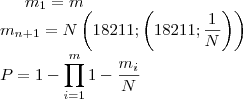# Rainbow Tables: Coverage

A rainbow table is generated by creating (m) chains using randomly picked starting keys. The reduction functions result (or ought to result at least) in evenly distributed new keys. Their is only a probability that a certain key/cipher pair is in the table.

I’ll gradually explain how the coverage can be calculated.

Take m chains, each 1 long to cover a keyspace of N keys. The chance that you can find the key is:When we have 1/2N chains, we’ll subsequently have a P of 1/2, a 50% coverage. Obvious.

When each chain would be 2 in length it gets trickier.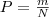still is the chance that we can find the key in the first column (first item of each chain of the table) of the table. The second column isn’t as straight forward. We’ve blindly assumed that each key in the first column is unique, which is true for the first column, but certainly isn’t for the second column. A few chains can merge. The second column is ‘random’.

The chance that a certain key is in a specific chain of the second column is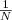. The chance that a certain key is in the second column is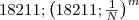(One minus the chance that there isn’t a certain key in a certain chain multiplied by itself the-amount-of-chains times).

The amount of keys covered by this column is the chance that a certain key is in the column times the amount of keys: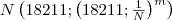.

The chance a certain key is in any of the two columns would be: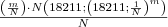The third column’s coverage can be calculated by taking the unique keys in the second column as m was taken for the amount of unique keys for the first column. With each chain t in length this formula applies: